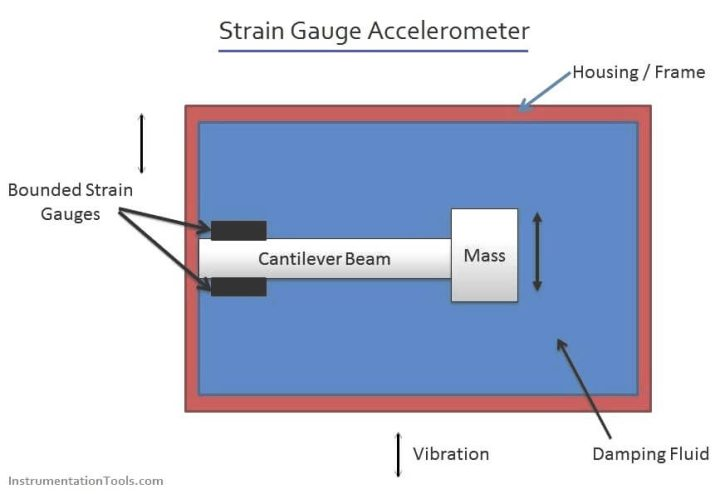# Strain Gauge Accelerometer

Principle of Strain Gauge Accelerometer

When a cantilever beam attached with a mass at its free end is subjected to vibration, vibrational displacement of the mass takes place. Depending on the displacement of the mass, the beam deflects and hence the beam is strained. The resulting strain is proportional to the vibration displacement of the mass and hence the vibration/acceleration being measured when calibrated.The main parts of a strain gauge accelerometer are as follows:

• A cantilever beam fixed to the housing of the instrument.
• A mass is fixed to the free end of the cantilever beam.
• Two bounded strain gauges are mounted on the cantilever beam as shown in diagram.
• Damping is provided by a viscous fluid filled inside the housing.

#### Operation of Strain Gauge Accelerometer

• The accelerometer is fitted on to the structure whose acceleration is to be measured.
• Due to the vibration, vibrational displacement of the mass occurs, causing the cantilever beam to be strained.
• Hence the strain gauges mounted on the cantilever beam are also strained and due to this their resistance change.
• Hence a measure of this change in resistance of the strain gauge becomes a measure of the extent to which the cantilever beam is strained.
• But the resulting strain of the cantilever beam is proportional to the vibration/acceleration and hence a measure of the change in resistance of the strain gauges becomes a measure of vibration/acceleration.
• The leads of the strain gauges are connected to a wheat stone bridge whose output is calibrated in terms of vibration/acceleration.